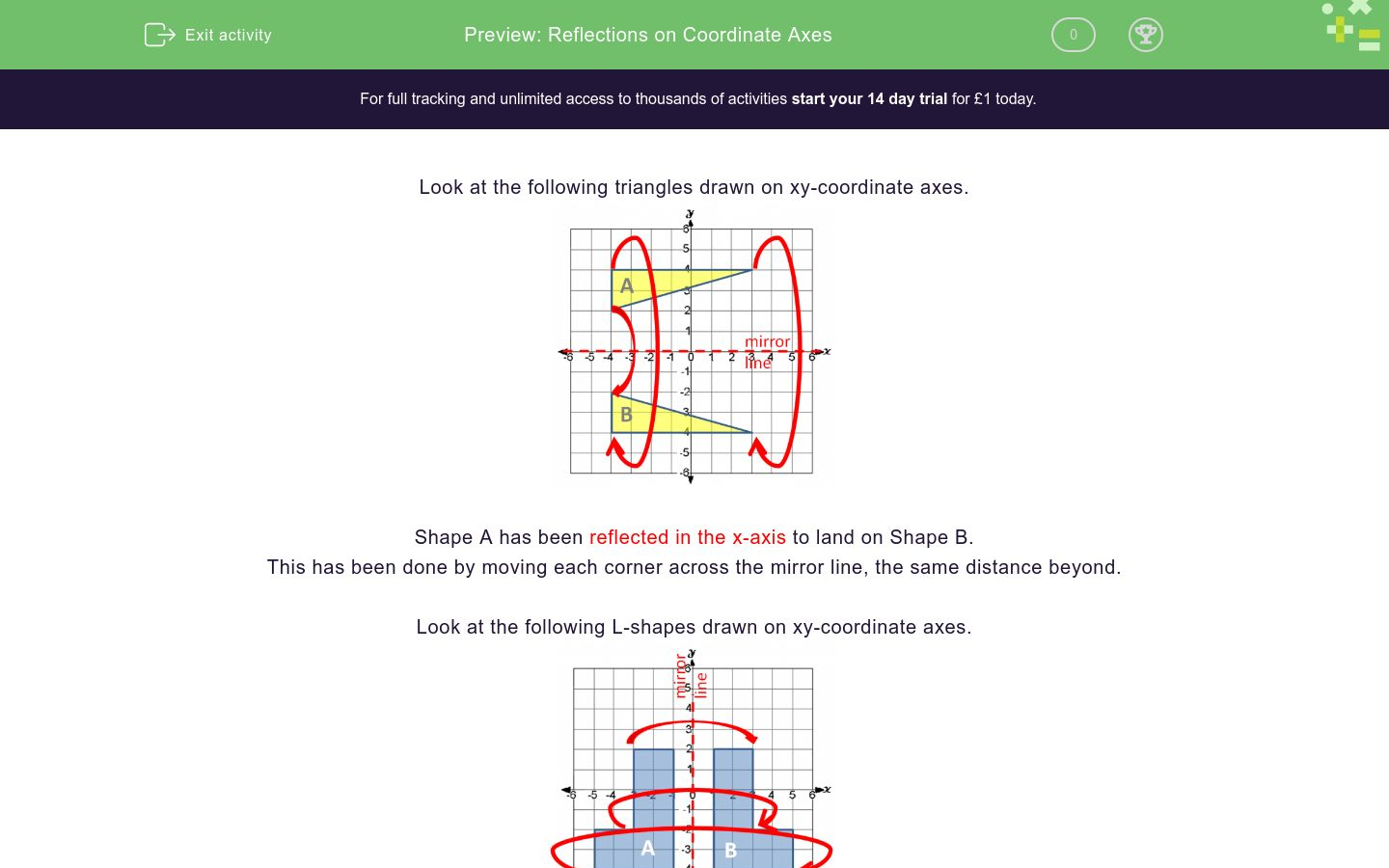# Reflections on Coordinate Axes

In this worksheet, students reflect shapes on a coordinate grid.Key stage:  KS 2

Curriculum topic:   Geometry: Position and Direction

Curriculum subtopic:   Draw/Translate Shapes

Difficulty level:### QUESTION 1 of 10

Look at the following triangles drawn on xy-coordinate axes.Shape A has been reflected in the x-axis to land on Shape B.

This has been done by moving each corner across the mirror line, the same distance beyond.

Look at the following L-shapes drawn on xy-coordinate axes.Shape A has been reflected in the y-axis to land on Shape B.

This has been done by moving each corner across the mirror line, the same distance beyond.

Example

Look at the triangles on this coordinate grid.

On which triangle does Triangle D land when it is reflected in the y-axis?Triangle D lands on Triangle A when it is reflected in the y-axis.

NB Triangle D lands on Triangle K when it is reflected in the x-axis.

Look at the triangles on this coordinate grid.

On which triangle does Triangle A land when it is reflected in the y-axis?A

B

C

D

E

F

G

H

I

J

K

none of them

Look at the triangles on this coordinate grid.

On which triangle does Triangle C land when it is reflected in the y-axis?A

B

C

D

E

F

G

H

I

J

K

none of them

Look at the triangles on this coordinate grid.

On which triangle does Triangle A land when it is reflected in the x-axis?A

B

C

D

E

F

G

H

I

J

K

none of them

Look at the triangles on this coordinate grid.

On which triangle does Triangle H land when it is reflected in the x-axis?A

B

C

D

E

F

G

H

I

J

K

none of them

Look at the triangles on this coordinate grid.

On which triangle does Triangle F land when it is reflected in the y-axis?A

B

C

D

E

F

G

H

I

J

K

none of them

Look at the triangles on this coordinate grid.

On which triangle does Triangle K land when it is reflected in the x-axis?A

B

C

D

E

F

G

H

I

J

K

none of them

Look at the triangles on this coordinate grid.

On which triangle does Triangle C land when it is reflected in the x-axis?A

B

C

D

E

F

G

H

I

J

K

none of them

Look at the triangles on this coordinate grid.

On which triangle does Triangle B land when it is reflected in the x-axis?A

B

C

D

E

F

G

H

I

J

K

none of them

Look at the triangles on this coordinate grid.

On which triangle does Triangle G land when it is reflected in the y-axis?A

B

C

D

E

F

G

H

I

J

K

none of them

Look at the triangles on this coordinate grid.

On which triangle does Triangle E land when it is reflected in the y-axis?A

B

C

D

E

F

G

H

I

J

K

none of them

• Question 1

Look at the triangles on this coordinate grid.

On which triangle does Triangle A land when it is reflected in the y-axis?D
EDDIE SAYS
A flips over the y-axis to land on D.
• Question 2

Look at the triangles on this coordinate grid.

On which triangle does Triangle C land when it is reflected in the y-axis?B
EDDIE SAYS
C flips over the y-axis to land on B.
• Question 3

Look at the triangles on this coordinate grid.

On which triangle does Triangle A land when it is reflected in the x-axis?H
EDDIE SAYS
A flips over the x-axis to land on H.
• Question 4

Look at the triangles on this coordinate grid.

On which triangle does Triangle H land when it is reflected in the x-axis?A
EDDIE SAYS
H flips over the x-axis to land on A.
• Question 5

Look at the triangles on this coordinate grid.

On which triangle does Triangle F land when it is reflected in the y-axis?G
EDDIE SAYS
F flips over the y-axis to land on G.
• Question 6

Look at the triangles on this coordinate grid.

On which triangle does Triangle K land when it is reflected in the x-axis?D
EDDIE SAYS
K flips over the x-axis to land on D.
• Question 7

Look at the triangles on this coordinate grid.

On which triangle does Triangle C land when it is reflected in the x-axis?none of them
EDDIE SAYS
C flips over the y-axis to land on B, but when flipped over the x-axis there is no matching triangle.
• Question 8

Look at the triangles on this coordinate grid.

On which triangle does Triangle B land when it is reflected in the x-axis?I
EDDIE SAYS
B flips over the x-axis to land on I.
• Question 9

Look at the triangles on this coordinate grid.

On which triangle does Triangle G land when it is reflected in the y-axis?F
EDDIE SAYS
G flips over the y-axis to land on F.
• Question 10

Look at the triangles on this coordinate grid.

On which triangle does Triangle E land when it is reflected in the y-axis?none of them
EDDIE SAYS
When E is reflected in the y-axis there is no matching shape.
---- OR ----